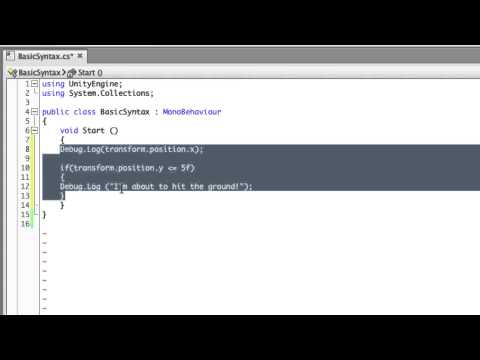Conventions and Syntax

Сложность: Базовая

Learn about some basic conventions and syntax of writing code - dot operators, semi-colons, indentation and commenting.Conventions and Syntax

Базовая Scripting

Code snippet

``````using UnityEngine;
using System.Collections;

public class BasicSyntax : MonoBehaviour
{
void Start ()
{
Debug.Log(transform.position.x);

if(transform.position.y <= 5f)
{
Debug.Log ("I'm about to hit the ground!");
}
}
}``````
``````#pragma strict

function Start ()
{
Debug.Log(transform.position.x);

if(transform.position.y <= 5f)
{
Debug.Log ("I'm about to hit the ground!");
}
}``````
``````import UnityEngine
import System.Collections

public class BasicSyntax(MonoBehaviour):

private def Start():
Debug.Log(transform.position.x)

if transform.position.y <= 5.0F:
Debug.Log('I\'m about to hit the ground!')``````